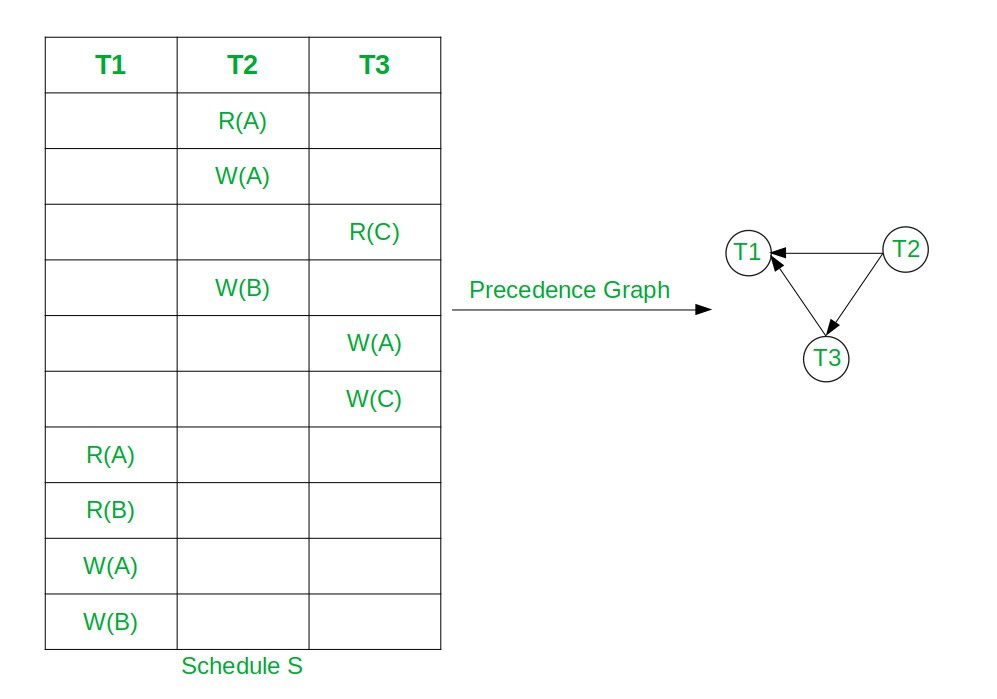Skip to content
Related Articles
Equivalent Serial Schedule of Conflict Serializable Schedule in DBMS
• Difficulty Level : Hard
• Last Updated : 12 Mar, 2021

Prerequisite: Conflict Serializability, Precedence Graph

Conflict Serializable Schedule: A schedule is called conflict serializable if it can be transformed into a serial schedule by swapping non-conflicting operations. The serial schedule of the Conflict Serializable Schedule can be found by applying topological Sorting on the Precedence Graph of the Conflict Serializable Schedule.

Note: Precedence Graph of Conflict Serial Schedule is always directed acyclic graph.

Approach: Follow to below steps to find topological sorting of Precedence Graph:

• Find the indegree of all nodes for the given Precedence Graph and store it in an auxiliary array.
• Now For each node having indegree 0 perform the following:
• Print the current node T as the order of the topological sort.
• Let the node T be the node with in-degree 0.
• Remove T and all edges connecting to T from the graph.
• Update indegree of all nodes after the above steps.
• After the above steps, the topological sort of the given precedence graph can be calculated.

Below is the illustration of the above approach:

Let, the Conflict Serial Schedule be S: R2(A) W2(A) R3(C) W2(B) W3(A) W3(C) R1(A) R2(B) W1(A) W2(B)• Here node T2 has indegree 0.
• So, select T2 and remove T2 and all edges connecting from it.
• Now T3 has indegree 0. So, select T3 and remove the edge T3→T1.
• At the end select T3. So the topological Sorting is T2, T3, T1.
• Hence, the equivalent serial schedule of given conflict serializable schedule is T2→T3→T1, i.e., S2: R2(A) W2(A) W2(B) R3(C) W3(A) W3(C) R1(A) R2(B) W1(A) W1(B).

There may be more than one equivalent serial schedule of the conflict serializable schedule.

Below is the implementation to get the Serial Schedule of CSS using Topological Sorting:

## C++

 `// C++ program to print serial schedule``// of CSS using Topological sorting``#include ``using` `namespace` `std;`` ` `class` `PrecedenceGraph {`` ` `    ``// No. of vertices``    ``int` `V;`` ` `    ``// Pointer to an array containing``    ``// adjacency vector``    ``vector<``int``>* adj;`` ` `    ``// Vector to store SerialSchedule``    ``vector<``int``> serialSchedule;`` ` `    ``// Function  declarations``    ``void` `computeIndegree(``int``* indegree);``    ``int` `findZeroIndegree(``int``* indegree,``                         ``bool``* visited);`` ` `public``:``    ``// Constructor``    ``PrecedenceGraph(``int` `V);`` ` `    ``// Function declarations``    ``void` `addEdge(``int` `v, ``int` `w);``    ``void` `topologicalSort();``    ``void` `printSchedule();``};`` ` `// Function to create the precedence``// graph``PrecedenceGraph::PrecedenceGraph(``int` `V)``{``    ``this``->V = V;``    ``adj = ``new` `vector<``int``>[V];``}`` ` `// Function to add the edge to the``// precedence graph``void` `PrecedenceGraph::addEdge(``int` `v,``                              ``int` `w)``{``    ``adj[v].push_back(w);``}`` ` `// Funciton to compute indegree of nodes``void` `PrecedenceGraph::computeIndegree(``    ``int``* indegree)``{``    ``for` `(``int` `i = 1; i < V; i++) {`` ` `        ``// Traverse the adjacency list``        ``// of node i``        ``for` `(``int` `j = 0;``             ``j < adj[i].size(); j++) {``            ``int` `node = adj[i][j];`` ` `            ``// Update the indegree``            ``// of node``            ``indegree[node]++;``        ``}``    ``}``}`` ` `// Function to find node with``// zero indegree``int` `PrecedenceGraph::findZeroIndegree(``    ``int``* indegree, ``bool``* visited)``{``    ``for` `(``int` `i = 1; i < V; i++) {`` ` `        ``// If node is not visited``        ``// and have indegree 0``        ``if` `(!visited[i]``            ``&& indegree[i] == 0) {`` ` `            ``// Mark node visited``            ``visited[i] = ``true``;`` ` `            ``// Return node``            ``return` `i;``        ``}``    ``}`` ` `    ``// All nodes are visited``    ``return` `-1;``}`` ` `// Function to find the topological``// Sorting of the given graph``void` `PrecedenceGraph::topologicalSort()``{``    ``// Create and initialize``    ``// visited array``    ``bool``* visited = ``new` `bool``[V]();`` ` `    ``// Create and initialize``    ``// indegree array``    ``int``* indegree = ``new` `int``[V]();`` ` `    ``computeIndegree(indegree);`` ` `    ``// Check if the node with``    ``// indegree 0 is available``    ``int` `node = findZeroIndegree(``        ``indegree, visited);`` ` `    ``bool` `nodeAvailable = ``false``;`` ` `    ``if` `(node != -1) {``        ``nodeAvailable = ``true``;``    ``}``    ``while` `(nodeAvailable) {`` ` `        ``// Add node to serial schedule``        ``serialSchedule.push_back(node);`` ` `        ``for` `(``int` `i = 0;``             ``i < adj[node].size(); i++) {`` ` `            ``// Delete all edges of current``            ``// node and update indegree``            ``indegree[adj[node][i]]--;``        ``}`` ` `        ``// Find next node with indegree 0``        ``node = findZeroIndegree(indegree,``                                ``visited);`` ` `        ``if` `(node == -1) {`` ` `            ``// Node with zero indegree``            ``// not available``            ``nodeAvailable = ``false``;``        ``}``    ``}``}`` ` `// Function to print the serial schedule``void` `PrecedenceGraph::printSchedule()``{``    ``for` `(``int` `i : serialSchedule) {``        ``cout << ``"T"` `<< i << ``" "``;``    ``}``}`` ` `// Driver Code``int` `main()``{``    ``// Create a precedence graph``    ``// given in the above diagram``    ``PrecedenceGraph graph(4);``    ``graph.addEdge(2, 1);``    ``graph.addEdge(2, 3);``    ``graph.addEdge(3, 1);`` ` `    ``// Find topological ordereing``    ``graph.topologicalSort();`` ` `    ``// Print Schedule``    ``cout << ``"Equivalent Serial"``         ``<< ``" Schedule is :"``;``    ``graph.printSchedule();``}`
Output:
```Equivalent Serial Schedule is :T2 T3 T1
```

Time Complexity: O(N)
Auxiliary Space: O(N)

Attention reader! Don’t stop learning now. Get hold of all the important DSA concepts with the DSA Self Paced Course at a student-friendly price and become industry ready.  To complete your preparation from learning a language to DS Algo and many more,  please refer Complete Interview Preparation Course.

In case you wish to attend live classes with industry experts, please refer Geeks Classes Live

My Personal Notes arrow_drop_up